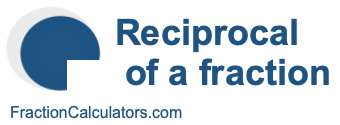Reciprocal of 3/9What is the reciprocal of 3/9? Here we will define reciprocal of 3/9, and show you how to calculate the reciprocal of 3/9 in fraction form and decimal form.

The reciprocal of 3/9 is a fraction or a number that when multiplied by 3/9 is equal to 1. To get the reciprocal of 3/9 based on that definition, we can make the following equation where "R" is the reciprocal of 3/9.

(3/9) × R = 1

When we solve for R, we get the answer to the reciprocal of 3/9 as a fraction as follows:

(3/9) × R = 1
R = 9/3
Reciprocal of 3/9 = 9/3

You can check that the answer is correct by confirming that 3/9 times the reciprocal of 3/9 is equal to 1, like this:

3/9 × 9/3 = 1

To find the decimal answer to the reciprocal of 3/9, you divide the numerator of the reciprocal by the demoninator of the reciprocal, like this:

9 ÷ 3 = 3
Reciprocal of 3/9 = 3

Tip: As you may have inferred from our tutorial above, you can quickly get the reciprocal of any fraction, such as 3/9, by switching the numerator and the denominator.

Reciprocal of a fraction
Do you need the reciprocal of another fraction? No problem! Please enter another fraction below.

Reciprocal of /

Reciprocal of 3/10
Here is the next fraction on our list that we have calculated the reciprocal of.